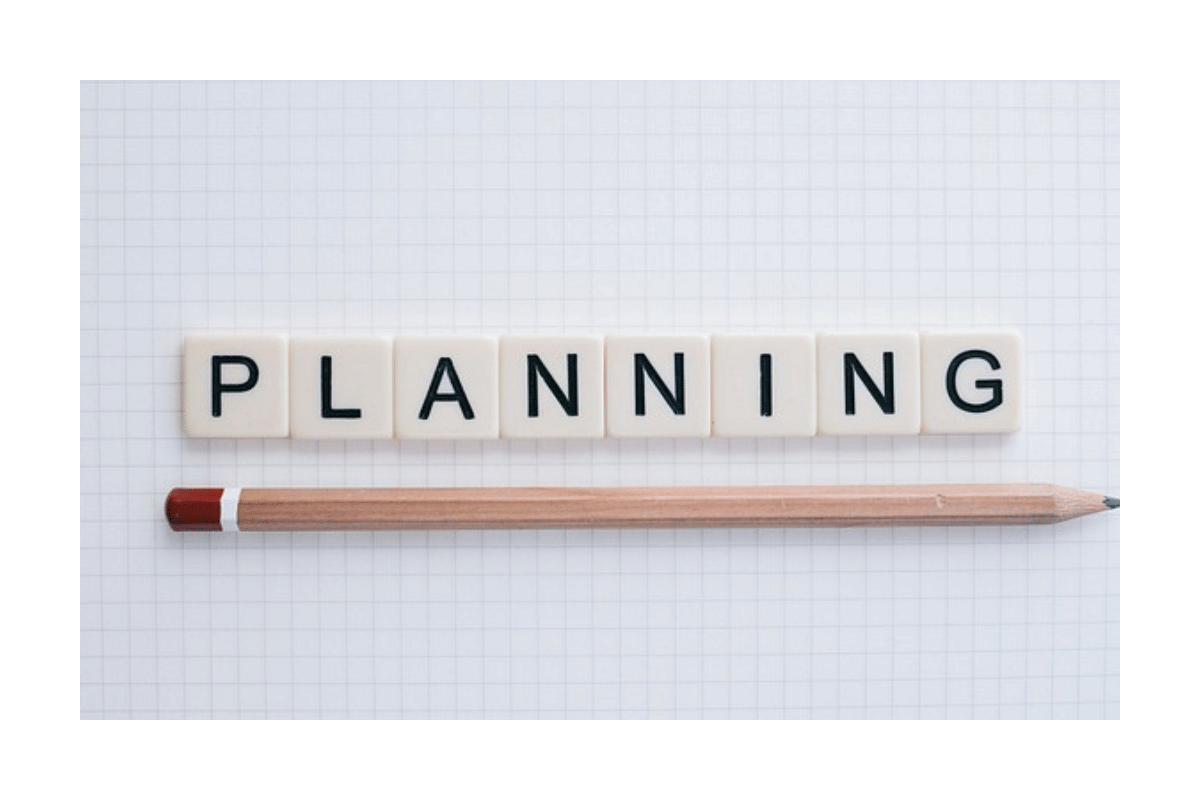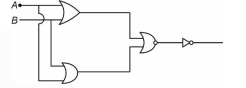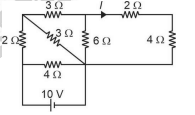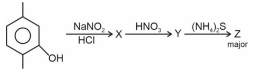# JEE Main 2024 Daily Practice Questions for 13 September 2023

Here the candidates will find the list of questions from Physics, Chemistry, and Mathematics separately so that they can start solving those questions from now onwards to secure a good rank in the JEE Main 2024 exam.JEE Main 2024 Daily Practice Questions for 13 September 2023

JEE Main 2024 Daily Practice Questions: As per the expectation, JEE Main 2024 session 1 exam will be conducted on January 2024 (considering the previous years’ trends). Hence, it is necessary to start practicing the daily JEE Main question papers to get a good score in the exam. Candidates should give equal importance to Physics, Chemistry, and Mathematics. Consistent practice is very useful to secure a high rank in the JEE Main exam.

# JEE Main 2024 Daily Practice Questions

Find out the JEE Main 2024 daily practice questions on Physics, Chemistry and MAthematics here in the given section-

### Physics:

Ques. The resultant gate is-1. NAND

2. NOR

3. OR

4. AND

Ques. For the given circuit diagram, find the current l.1. (5/16)A

2. (5/48)A

3. (5/12)A

4. (1/16)A

Ques. A source of sound is moving away from a stationary observer with a constant velocity of 40 m/s. Find the frequency heard by the observer, if the original frequency of the source is 400 Hz and the speed of sound in the air is 360 m/s

1. 330 Hz

2. 320 Hz

3. 360 Hz

4. 280 Hz

### Chemistry:

Ques. Which of the following has a minimum boiling point?

1. Na

2. K

3. Rb

4. Cs

Ques. Red ppt. by Benedict solution is-

1. Glucose

2. RNA
3. DNA

4. Sucrose

Ques.Compound Z is.

### Mathematics:

Ques. 5 boys with allotted roll numbers and seat numbers are seated in such a way that no one sits on the allotted seat. Find the number of such seating arrangements.

1. 18

2. 9

3. 45

4. 44

Ques. In the expansion of (x+2)9 the mean of coefficient of X, X2, X3,....,x9 is:

1. 38/10

2. 36/10

3. 37/5

4. 39/10

Ques. If x+y+z = 17 and x, y, z are non-negative integers, then find the number of integral solutions is-

1. 136

2. 271

3. 90

4. 130

Stay tuned to CollegeDekho for more Education News pertaining to entrance exams and admission. You can also write to us at our E-Mail ID news@collegedekho.com.

/news/jee-main-2024-daily-practice-questions-for-13-september-2023-45046/

### Do you have a question? Ask us.

• Typical response between 24-48 hours

• Get personalized response

• Free of Cost